If you have a related question, please click the "Ask a related question" button in the top right corner. The newly created question will be automatically linked to this question.

# BOOSTXL-DRV8305EVM: BLDC motor control

Part Number: BOOSTXL-DRV8305EVM
Other Parts Discussed in Thread: DRV8305

Good, morning,

I am trying to control a BLDC motor using an Arduino Due board combined with your motor driver BOOSTXL-DRV8305EVM in 1-PWM mode, but I had some troubles. At first, once set the PWM duty cycle value, the motor doesn't start by itself but it is necessary to give a manual "input" (rotating it manually) until it begins the rotation. This is the first issue. Furthermore, some problems rise with the PWM duty cycle value. Indeed:

INPUT Voltage= 24 V,  duty_cycle=0.8 --> effective_Voltage= 24*0.8= 19.2 V --> motor doesn't start and the driver gives fault

INPUT Voltage= 30 V,  duty_cycle=0.65 --> effective_Voltage= 24*0.8= 19.5 V --> motor starts rotating

Hence, I assume that the problem is related to the duty cycle value given as input to the driver but i cannot understand why this happens.

I attach also the code used to generate the PWM signals according to the hall sensors output

```function [A,B,C,D,Enable,Duty_cycle] = fcn(H1,H2,H3,Duty_cycle)
A=0; %First MCU PWM output
B=0; %Second MCU PWM output
C=0; %Third MCU PWM output
D=0;
Enable=0;
Duty_cycle=Duty_cycle %set on the Init code and kept constant initially

if H1==0 & H2==1 & H3==1
A=0;
B=1;
C=0;
elseif H1==0 & H2==1 & H3==0
A=1;
B=1;
C=0;
elseif H1==1 & H2==1 & H3==0
A=1;
B=0;
C=0;
elseif H1==1 & H2==0 & H3==0
A=1;
B=0;
C=1;
elseif H1==1 & H2==0 & H3==1
A=0;
B=0;
C=1;
elseif H1==0 & H2==0 & H3==1
A=0;
B=1;
C=1;
end
end

```

Regards,

Simone Mantica

• Hello Simone,

Just to confirm, have you checked the connections and firmware of https://www.ti.com/tool/TIDA-00645?

What are the specifications of the motor that you are using?

Thanks,

Matt

• Yes, I have checked the hall sensors outputs using the oscilloscope and they behave correctly. Indeed, after moving the motor manually, as in the attached video, it starts rotating correctly for proper duty cycle values (the noise is related to the support vibrations, not to the motor itself)

About the motor spec., they' re the following ones: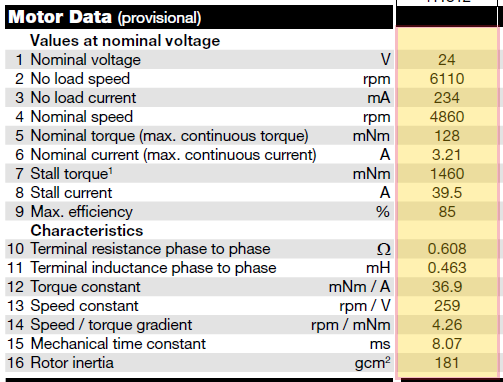Here it is the link of the video:

www.dropbox.com/.../VID_20210907_144811.mp4

• Hello Simone,

Thanks for the motor info. I believe the issue might stem directly from the code. Is this function being looped continuously by the MCU?

Is there a way you can rearrange the wires from the hall sensor to match the outputs from your MCU (Outputs A, B, C)?

If the current vector is either 0 or not pointing in the right direction at startup then this might be why the motor is not starting by itself.

Best,

Isaac

• Yes this function is being looped continuosly by the MCU. It reads the hall sensors' outputs and then send the a signal to the motor driver with the next combination for the motor's phases. I suppose it maybe a fixed initial delay or something similar related to the code execution by the MCU.

However I still have the initial problem related to the duty cycle values. Indeed the driver gives an error and the motor stops rotating as i explained in the initial question.

Thanks,

Simone

• Hello Simon,

Sorry I must have misunderstood the question, I was not aware the motor would stop turning after it started, I was under the impression that the motor would not start by itself.

Is this condition the one in which the motor starts but stops after the fault?

INPUT Voltage= 30 V,  duty_cycle=0.65 --> effective_Voltage= 24*0.8= 19.5 V --> motor starts rotating

What fault is being given by the device? And what are your current IDRIVE settings?

Best,

Isaac

• The device gives me the PVDD undervoltage 2 fault and the current IDRIVE settings are the predefined ones. Yes, my problem is that the motor for some duty cycle value doesn't start by itself.

• Hello Simone,

The PVDD_UVLO2 fault indicates that your PVDD voltage is too low, ~4.4V. When this occurs your gate drive outputs will be pulled low and the gate drive supplies will be disabled making you unable to drive the motor. If you turn the motor then this could create some voltage on the supply, getting you out of the PVDD_UVLO2 fault and giving you the ability to drive the motor. Have you probed PVDD to confirm the voltage is as you expected?

Best,

Isaac

• I've realized that the starting delay was related to the code, but i still i have the undervoltage problem. My power supply voltage is 24 V linked to the driver. Firstly, before giving the PWM signal, I have read with the oscilloscope VPVDD and it was a bit less than 2 V. Then, when PWM signal starts, the PVDD goes to zero (but i don't know why) and the driver gives me UNDERVOLTAGE ERROR. In this case the motor does not rotate.

Furthermore, during a second attempt by turning off and then on the power supply, the motor starts rotating by itself (producing a strange noise), but the PVDD still mantains at zero.

I've also measured the output voltage of the driver to the motor windings once the pwm signal started: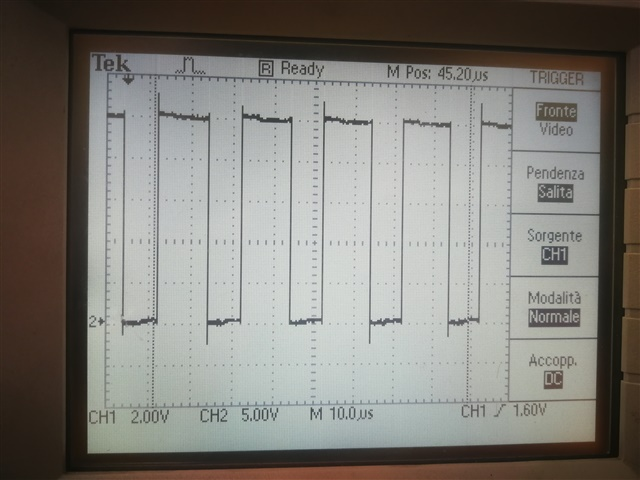This is the output from MOTB (fixed at zero; before starting the PWM, during the driver setup phase it is fixed at 24 V more or less so the problem is not the wire connection)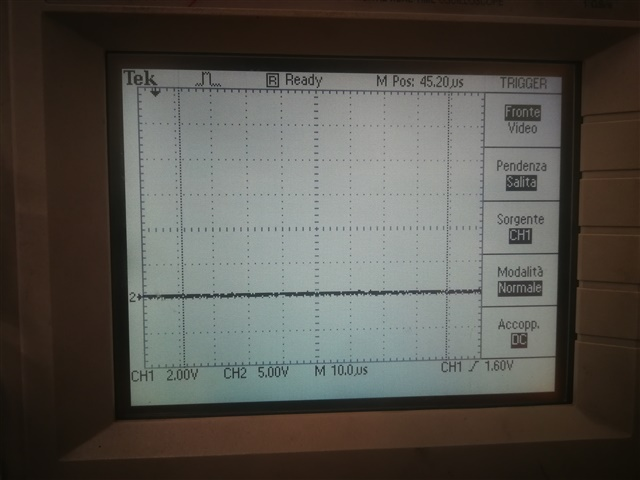This is the output from MOTC (fixed at 24 V; during the driver setup phase it is fixed at 24 V more or less)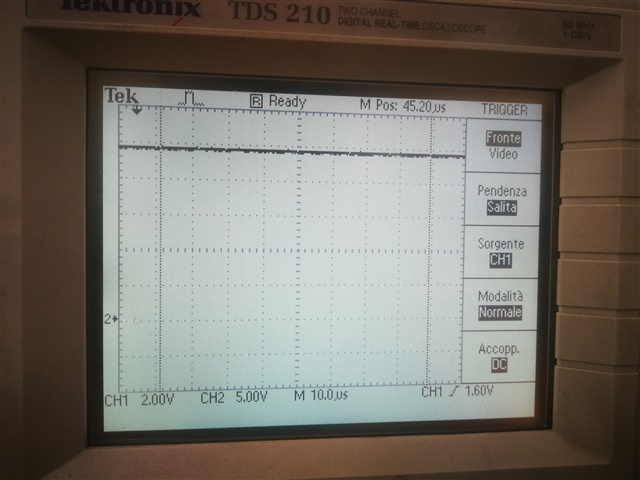Then while the motor rotates, according to the supplied phase I checked that they switch correctly.

But I don't understand why the outputs are these ones, since I expect to have three oscillating signals between -24 and +24 V as in the following picture (with an inversion of polarity, that seems not to be present in my case):• Hello Simone,

So it seems like the PVDD voltage is fine some of the time (based on your scope captures) are  there any specific conditions that you are doing differently when the device gives you the UVLO error? What is the current limit you have set on your supply?

If the MCU is not being turned off then the code could most likely still be running and it could still be attempting to drive the motor, as far as the audible noise that you are hearing is most likely because the motor is spinning at a slow speed, which is below 20kHz so this becomes audible to the human hearing range. Typically during motor operation it is spinning faster than this hence why you cant hear it, this is a pretty common issue when spinning motors slowly though. As far as the image goes, this image is not correct. You do not need a positive and negative supply in order to change the polarity to commutate the motor, I believe the label here is supposed to be phase and not voltage. I recommend you to watch this video on trapezoidal commutation: https://training.ti.com/ti-precision-labs-motor-drives-commutation-trapezoidal

Best,

Isaac

• Hi Isaac,

Thanks for the answer about the motor phase supplying, now I've understood. However i have the PVDD undervoltage issue.

are  there any specific conditions that you are doing differently when the device gives you the UVLO error?

No, there is not any different condition, it gives me the error always when the PWM signal starts .

Nevertheless, this morning I have done the same operations with MOTC not connected for mistake (I have not noticed that ONLY MOTA and MOTB where connected) and the motor starts rotating normally (a bit noisy due to the fact that only two phases were connected, I suppose). Then, correcting the error and connecting MOTC, again the motor does not start and PVDD undervoltage error occurs. Thus, I've tried also different "experiments" by connecting only MOTA and MOTC or only MOTB and MOTC and the result is the same: when two phases are connected it seems to work correctly, plugging the third phase wire the driver returns the undervoltage error and enters in standby.

So it seems like the PVDD voltage is fine some of the time (based on your scope captures

I think I have not understand properly what is PVDD. Reading from the datasheet, I suppose it was the driver supply voltage, not the motor windings' one, is it true or not? Furthermore why measuring it from VSEN PVDD it is initially more or less 2V during driver setting up phase while when PWM starts it goes to zero?

What is the current limit you have set on your supply?

It is 1.95 A, so I think it is a quite reasonable value for the current limit (considering that right now the motor has no load)

Another issue that I have is related to the big electric noise entering in the driver from the power supply, which has not a good internal filter to reduce it. May the undervoltage fault be related even to this?

• Hello Simone,

Yes PVDD is the from the driver voltage supply, VSEN_PVDD comes from a voltage sense circuit which is connected directly to the voltage supply (image below) but if you notice there is a voltage divider along with a filter cap that connects to the MCU.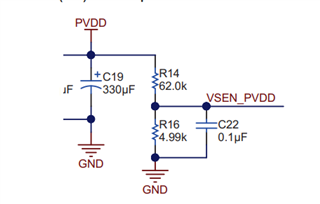While this does give you somewhat of an indicator of what is going on with PVDD I would recommend checking your actual supply to make sure that there is nothing actually changing on the supply. Also note that upon power up the device could give you a UVLO fault, once this is cleared does it come back again while you are trying to run the motor? The VSEN_PVDD could be showing 2V because of the added circuit so it is best to check what the true value of PVDD is and make sure that is not undervoltage.

The current limit seems fine, but that mainly depends on the motor that you are driving. I have not encountered this but if the power supply is noisy it might  be possible for the device is giving you an undervoltage fault. VSEN_PVDD is sampled by an ADC on the launchpad if this is really noisy then its possible that it is sampling incorrectly and giving you the fault. Not sure if this is actually happening but just a thought. I would monitor the supply to check how it looks when trying to drive the motor and make sure that it does not go into an actual undervoltage condition.

Best,

Isaac

• Hello Isaac,

I have checked the power supply behavior with the oscilloscope when the driver returns the error and it keeps constant at 24 V. Hence, I suppose the problem linked to the ADC which achieve a wrong measurement causing the fault.

Also note that upon power up the device could give you a UVLO fault, once this is cleared does it come back again while you are trying to run the motor?

It depends on the situation, in some case it happens again, in particular when i try to change the duty cycle value to control the speed.

Furthermore, I have noticed that the same error occurs when I try to stop the motor in a prescriped position. Indeed I use the following code through which, when the motor reaches a precise angle (measured by the encoder), I give the command to stop it by keeping powering the current phase without any switching:

```if H1==0 & H2==1 & H3==1
if angle>=angle_ref
A=0;
B=1;
C=1;

else
A=0;
B=1;
C=0;
end
elseif H1==0 & H2==1 & H3==0
if angle>=angle_ref
A=0;
B=1;
C=0;

else
A=1;
B=1;
C=0;
end
elseif H1==1 & H2==1 & H3==0
if angle>=angle_ref
A=1;
B=1;
C=0;

else
A=1;
B=0;
C=0;
end
elseif H1==1 & H2==0 & H3==0
if angle>=angle_ref
A=1;
B=0;
C=0;

else
A=1;
B=0;
C=1;
end
elseif H1==1 & H2==0 & H3==1
if angle>=angle_ref
A=1;
B=0;
C=1;
%Duty_cycle=0;
else
A=0;
B=0;
C=1;
end
elseif H1==0 & H2==0 & H3==1
if angle>=angle_ref
A=0;
B=0;
C=1;

else
A=0;
B=1;
C=1;
end
end

end```

(H1,H2,H3 are the hall sensors output and A,B,C are the inputs from the MCU to the driver)

Is it correct? Must I also set the duty cycle equal to zero to stop the motor or do I have to consider the stop state from the truth table (0000)?

And if I want to let it restart rotating in opposite verse, is it sufficient to reverse the Hall sensor sequence from the truth table or do I have to do something else?

Best,

Simone

• Hello Simone,

Okay thanks for the info that explains a whole lot more. A couple of things to note here when you are changing the duty cycle you need to make sure that the change is not too drastic. If the duty cycle change goes from 0% to 50% you would need to slowly increase the duty cycle.

When stopping the motor at a specific location it is not recommended to do it in the same method that you are doing in this code snippet. Whenever you drive one phase of the motor with all the voltage then this causes stall current, so your supply is trying to provide about ~40A of current to the motor. You want to ideally decelerate and stop the motor and you can keep a very low duty cycle, the set it to 0 when the motor has not inertia to keep it from moving forward. Typically it is okay to align the motor in this way but you need to use a very low current when doing this to avoid problems with your supply.

And yes if you want to reverse direction you can just reverse the hall sensor sequence form the truth table. Based on what you sent here I am almost certain that you are dealing with some power supply issues, the way to know for sure is to probe both the supply and nFaul. You can trigger on it and based on the conditions you described you are probably going to see a change on your supply when doing one of these changes.

Best,

Isaac

• Hello Isaaac,

You want to ideally decelerate and stop the motor and you can keep a very low duty cycle, the set it to 0 when the motor has not inertia to keep it from moving forward.

Ok, I've understood. Hence, is your suggestion only to act on the duty cycle value to stop the motor by reducing its value gradually?

Moreover, I don't understand what are the command "Stop" and "Align" used for in  the truth table?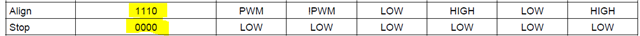Best,

Simone

• Hello Simone,

Yes you want to slow it down slowly, and either reduce the current to 0 or a very low current to hold it in position you want. Ideally you should stop driving the motor once it reaches the position you want to hold it.

The truth table you referenced here is used for the 1xPWM mode on the DRV8305. This mode intakes one PWM signal from your MCU in order to control the speed based on the duty cycle of the input PWM to INHA. The states control the output of the gates which are controlled by the input to INLA, INHB, and INLB. Your MCU basically directs which gates are driven based on the input state that it is presented with.

The align state is used to align the motor to determine the starting position of the motor, the reason this is helpful is because the motor will be in a known position at startup so this will help you determine how to start the commutation of the motor. You can watch this video for more information: https://training.ti.com/ti-precision-labs-motor-drivers-sensorless-startup-methods?context=1139747-1138777-1139742-1147202

The stop state just means the motor will not be driven in this state, and none of the GHx or GLx outputs will be active during this state.

Best,

Isaac